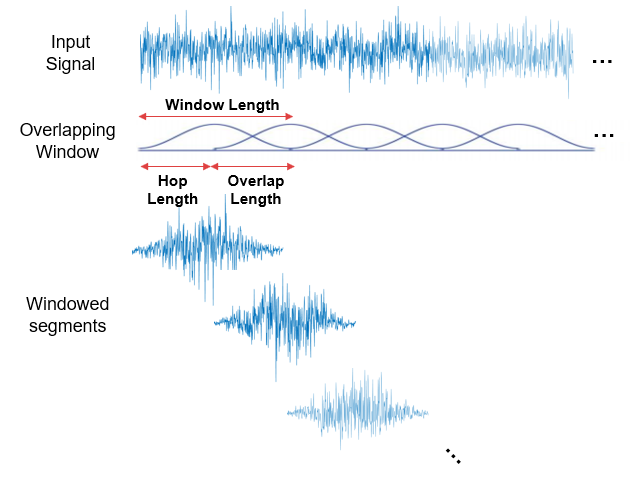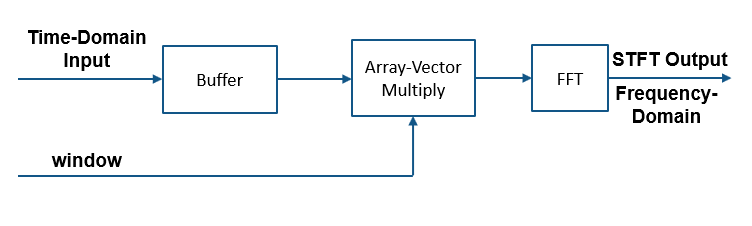# dsp.STFT

Short-time FFT

## Description

The `dsp.STFT` object computes the short-time Fourier transform (STFT) of the time-domain input signal. The object accepts frames of time-domain data, buffers them to the desired window length and overlap length, multiplies the samples by the window, and then performs FFT on the buffered windows. For more details, see Algorithms.

Use the STFT to analyze the frequency content of a signal that varies with time.

## Creation

### Syntax

``stf = dsp.STFT``
``stf = dsp.STFT(window)``
``stf = dsp.STFT(window,overlap)``
``stf = dsp.STFT(window,overlap,nfft)``
``stf = dsp.STFT(Name,Value)``

### Description

````stf = dsp.STFT` returns an object, `stf`, that implements the short-time FFT. The object processes the data independently across each input channel over time.```
````stf = dsp.STFT(window)` returns a short-time FFT object with the Window property set to `window`.```
````stf = dsp.STFT(window,overlap)` returns a short-time FFT object with the `Window` property set to `window` and the OverlapLength property set to `overlap`.```

example

````stf = dsp.STFT(window,overlap,nfft)` returns a short-time FFT object with the `Window` property set to `window`, the `OverlapLength` property set to `overlap`, and the FFTLength property set to `nfft`.```
````stf = dsp.STFT(Name,Value)` returns a short-time FFT object with each specified property name set to the specified value. You can specify additional name-value pair arguments in any order.```

## Properties

expand all

Analysis window, specified as a vector of real elements.

The object buffers the input into overlapping window segments using the specified window length and overlap length, and then multiplies each overlapped segment by the window.Tunable: Yes

Data Types: `single` | `double`

Number of samples by which consecutive windows overlap, specified as a positive integer. The window overlap reduces the artifacts at the data boundaries.Data Types: `single` | `double` | `int8` | `int16` | `int32` | `int64` | `uint8` | `uint16` | `uint32` | `uint64`

FFT length, specified as a positive integer. This property determines the length of the STFT output (number of rows). The FFT length must be greater than or equal to the window length.

Data Types: `single` | `double` | `int8` | `int16` | `int32` | `int64` | `uint8` | `uint16` | `uint32` | `uint64`

Frequency range over which the short-time FFT is computed, specified as:

• `'twosided'` ––The short-time FFT is computed for complex or real inputs signals. The length of the short-time FFT is equal to the value you specify in the `FFTLength` property.

• `'onesided'` –– The one-sided short-time FFT is computed for real input signals only. When the FFT length is even, the short-time FFT length is `FFTLength`/2+1. If FFT length is odd, the length of the short-time FFT is equal to (`FFTLength`+1)/2.

## Usage

### Syntax

``y = stf(x)``

### Description

example

````y = stf(x)` applies short-time FFT on the input `x` and returns the frequency-domain output `y`. ```

### Input Arguments

expand all

Time-domain input signal, specified as a vector or a matrix. If the input is a matrix, the object treats each column as an independent channel. The frame size (number of rows in `x`) must be equal to or less than the hop length (window length − overlap length).

The input can be a variable-sized signal. That is, the frame size of the signal can change in between calls to the object algorithm without calling the `release` function. The number of channels must remain the same.

If the `FrequencyRange` property is set to `'onesided'`, the input must be real. If the `FrequencyRange` property is set to `'twosided'`, the input can be real or complex.

Data Types: `single` | `double`
Complex Number Support: Yes

### Output Arguments

expand all

Short-time FFT output, returned as a vector or a matrix.

If there are enough samples (equal to hop length) to form an STFT output, `y` is an `FFTLength`-by-N matrix, where N is the number of input channels. If there are not enough samples to form an STFT output, `y` is empty.

The data type of the output matches that of the input signal.

Data Types: `single` | `double`
Complex Number Support: Yes

## Object Functions

 `step` Run System object algorithm `release` Release resources and allow changes to System object property values and input characteristics `reset` Reset internal states of System object `clone` Create duplicate System object `isLocked` Determine if System object is in use `getFrequencyVector` Get the vector of frequencies at which the short-time FFT is computed

## Examples

collapse all

Short-time spectral attenuation is achieved by applying a time-varying attenuation to the short-time spectrum of a noisy signal. The gain of the attenuation is determined by the estimate of the noise power in each subband of the spectrum. This gain, when applied to the noisy spectrum, attenuates the subbands with higher noise power and lifters the subbands with lesser noise power.

Here are the steps involved in performing the short-time spectral attenuation:

1. Analyze the noisy input signal by computing the short-time Fourier transform (STFT).

2. Multiply each subband of the transformed signal with a real positive gain less than 1.

3. Synthesize the denoised subbands by taking the inverse short-time Fourier transform (ISTFT). The resconstructed signal is the denoised input signal.

Use the `dsp.STFT` and `dsp.ISTFT` objects to compute the short-time and the inverse short-time Fourier transforms, respectively.

Noisy Input Signal

The input is an audio signal sampled at the 22,050 Hz. The `dsp.AudioFileReader` object reads this signal in frames of 512 samples. The audio signal is corrupted by white Gaussian noise that has a standard deviation of 0.05. Use the `audioDeviceWriter` object to play the noisy audio signal to your computer's audio device.

```FrameLength = 512; afr = dsp.AudioFileReader('speech_dft.wav',... 'SamplesPerFrame',FrameLength); adw = audioDeviceWriter('SampleRate',afr.SampleRate); noiseStd = 0.05; while ~isDone(afr) cleanAudio = afr(); noisyAudio = cleanAudio + noiseStd * randn(FrameLength,1); adw(noisyAudio); end reset(afr)```

Initialize Short-Time and Inverse Short-Time Fourier Transform Objects

Initialize the `dsp.STFT` and `dsp.ISTFT` objects. Set the window length equal to the input frame length and the hop length to 16. The overlap length is the difference between the window length and the hop length, OL = WLHL. Set the FFT length to 1024.

```WindowLength = FrameLength; HopLength = 16; numHopsPerFrame = FrameLength / 16; FFTLength = 1024;```

The window used to compute the STFT and ISTFT is a periodic `hamming` window with length 512. The `ConjugateSymmetricInput` flag of the `istf` object is set to `true`, indicating that the output of the `istf` object is a conjugate-symmetric signal.

```win = hamming(WindowLength,'periodic'); stf = dsp.STFT(win,WindowLength-HopLength,FFTLength); istf = dsp.ISTFT(win,WindowLength-HopLength,1,0);```

Gain Estimator

The next step is to define the gain estimator parameters. This gain is applied to the noisy spectrum to attenuate the subbands with higher noise power and lifter the subbands with lesser noise power.

```dec = 16; alpha = 15; stftNorm = (sum(win.*win) / dec).^2;```

Spectral Attenuation

Feed the audio signal to `stf` one hop-length at a time. Apply the estimated gain to the transformed signal. Reconstruct the denoised version of the original speech signal by performing an inverse Fourier transform on the individual frequency bands. Play the denoised audio signal to the computer's audio device.

```while ~isDone(afr) cleanAudio = afr(); noisyAudio = cleanAudio + noiseStd * randn(FrameLength,1); y = zeros(FrameLength,1); % y holds the denoised audio frame % Feed audio to stft one hop-length at a time for index = 1:numHopsPerFrame X = stf(noisyAudio((index-1)*HopLength+1:index*HopLength)); % Gain estimator Z = abs(X).^2 / (noiseStd^2 * alpha) / stftNorm; Z(Z<=1) = 1; Z = 1 - 1./Z; Z = sign(Z) .* sqrt(abs(Z)); X = X .* Z; % Convert back to time-domain y((index-1)*HopLength+1:index*HopLength) = istf(X); end % Listen to denoised audio: adw(y); end```

Perfect reconstruction is when the output of `dsp.ISTFT` matches the input to `dsp.STFT`. Perfect reconstruction is obtained if the analysis window, $\mathit{g}\left(\mathit{n}\right)$, obeys the constant overlap-add (COLA) property at hop-size R.

`$\sum _{\mathit{m}=-\infty }^{\infty }\mathit{g}\left(\mathit{n}-\mathit{mR}\right)=1,\text{\hspace{0.17em}}\forall \mathit{n}\in \text{\hspace{0.17em}}Ζ\text{\hspace{0.17em}\hspace{0.17em}\hspace{0.17em}\hspace{0.17em}\hspace{0.17em}}\left(\mathit{g}\in \mathrm{COLA}\left(\mathit{R}\right)\right)$`

A signal is perfectly reconstructed if the output of the `dsp.ISTFT` object matches the input to the `dsp.STFT `object.

`iscola` Function

The `iscola` function checks that the specified window and overlap satisfy the COLA constraint to ensure that the inverse short-time Fourier transform (ISTFT) results in perfect reconstruction for non-modified spectra. The function returns a logical `true` if the combination of input parameters is COLA-compliant and a logical `false` if not. The `method` argument of the function is set to `'ola'` or `'wola'` depending on whether the inversion method uses weighted overlap-add (WOLA).

Check if `hann()` window of length 120 samples and an overlap length of 60 samples is COLA compliant.

```winLen = 120; overlapLen = 60; win = hann(winLen,'periodic'); tf = iscola(win,overlapLen,'ola')```
```tf = logical 1 ```

Initialization

Initialize the `dsp.STFT` and `dsp.ISTFT` System objects with this `hann` window that is COLA compliant. Set the FFT length to equal the window length.

```frameLen = winLen-overlapLen; stf = dsp.STFT('Window',win,'OverlapLength',overlapLen,'FFTLength',winLen); istf = dsp.ISTFT('Window',win,'OverlapLength',overlapLen,'WeightedOverlapAdd',0);```

Reconstruct Data

Compute the STFT of a random signal. Set the length of the input signal to equal the hop length (window length – overlap length). Since the window is COLA compliant, the ISTFT of this non-modified spectra perfectly reconstructs the original time-domain signal.

To confirm, compare the input, x to the reconstructed output, y. Due to the latency introduced by the objects, the reconstructed output is shifted in time compared to the input. Therefore, to compare, take the norm of the difference between the reconstructed output, y and the previous input, xprev. The norm is very small, indicating that the output signal is a perfectly reconstructed version of the input signal.

```n = zeros(1,100); xprev = 0; for i = 1:100 x = randn(frameLen,1); X = stf(x); y = istf(X); n(1,i) = norm(y-xprev); xprev = x; end max(abs(n))```
```ans = 1.6972e-13 ```

In WOLA, a second window called the synthesis window, $\mathit{f}\left(\mathit{n}\right)$, is applied after the IFFT operation and before overlap-add. The synthesis and analysis windows are typically identical and are usually obtained by taking the square root of windows satisfying COLA (thereby ensuring perfect reconstruction).

`iscola` Function

Check if `sqrt(hann())` window of length 120 samples and an overlap length of 60 samples is WOLA compliant. Set the `method` argument of the `iscola` function to `'wola'`. The output of the `iscola` function is 1 indicating that this window is WOLA compliant.

```winWOLA = sqrt(hann(winLen,'periodic')); tfWOLA = iscola(winWOLA,overlapLen,'wola')```
```tfWOLA = logical 1 ```

Reconstruct Data with WOLA

Release the `dsp.STFT` and `dsp.ISTFT` System objects and set the window to `sqrt(hann(winLen,'periodic'))` window. To use weighted overlap-add on the `ISTFT` side, set the `'WeightedOverlapAdd'` to `true`.

```release(stf); release(istf); stf.Window = winWOLA; istf.Window = winWOLA; istf.WeightedOverlapAdd = true; n = zeros(1,100); xprev = 0; for i = 1:100 x = randn(frameLen,1); X = stf(x); y = istf(X); n(1,i) = norm(y-xprev); xprev = x; end max(abs(n))```
```ans = 4.6664e-15 ```

The `norm` of the difference between the input signal and the reconstructed signal is very small indicating that the signal has been reconstructed perfectly.

expand all

## Algorithms

Here is a sketch of how the algorithm is implemented:The time-domain input signal is buffered based on a user-specified window length (WL) and overlap length (OL). The hop size, R, is defined as R = WLOL. Buffered windows are multiplied by a user-specified window of length WL. The STFT output is the FFT of this product. The number of time-domain samples required to form a new FFT output is R.

Here is an illustration of how a random signal looks like in the original time-domain, after multiplying with the overlapping windows, and after applying FFT on the multiplied windows:Allen, J.B., and L. R. Rabiner. "A Unified Approach to Short-Time Fourier Analysis and Synthesis,'' Proceedings of the IEEE, Vol. 65, pp. 1558–1564, Nov. 1977.